## Main.DataSimulation History

April 28, 2019, at 05:44 AM by 45.56.3.173 -
Changed lines 68-69 from:

(:toggle hide gekko_mimo button show="Python TCLab MIMO ID":) (:div id=gekko_mimo:)

to:

(:toggle hide gekko_siso button show="Python TCLab SISO ID":) (:div id=gekko_siso:)

April 28, 2019, at 05:43 AM by 45.56.3.173 -
Changed line 95 from:

plt.ylabel('MV')

to:

plt.ylabel('MV Voltage (mV)')

Changed line 100 from:

plt.ylabel('CV')

to:

plt.ylabel('CV Temp (degF)')

Changed line 136 from:

plt.ylabel('MV')

to:

plt.ylabel('MV Voltage (mV)')

Changed line 141 from:

plt.ylabel('CV')

to:

plt.ylabel('CV Temp (degF)')

Changed lines 172-173 from:

plt.plot(m.time,yc.value,'b--',label=r'$T_1$') plt.ylabel('Temperature (K)')

to:

plt.plot(m.time,yc.value,'r--',label=r'$T_1$') plt.ylabel('Temperature (degF)')

April 28, 2019, at 05:38 AM by 45.56.3.173 -

Changed lines 64-182 from:

with p=2 (number of parameters), n=851 (number of measurements), \theta=[K,\tau] (parameters), \theta^* as the optimal parameters, SSE as the sum of squared errors, and the F statistic that has 3 arguments (alpha=confidence level, degrees of freedom 1, and degrees of freedom 2). For many problems, this creates a multi-dimensional nonlinear confidence region. In the case of 2 parameters, the nonlinear confidence region is a 2-dimensional space.

to:

with p=2 (number of parameters), n=851 (number of measurements), \theta=[K,\tau] (parameters), \theta^* as the optimal parameters, SSE as the sum of squared errors, and the F statistic that has 3 arguments (alpha=confidence level, degrees of freedom 1, and degrees of freedom 2). For many problems, this creates a multi-dimensional nonlinear confidence region. In the case of 2 parameters, the nonlinear confidence region is a 2-dimensional space.

#### Solution (GEKKO Python)

(:toggle hide gekko_mimo button show="Python TCLab MIMO ID":) (:div id=gekko_mimo:) (:source lang=python:) from gekko import GEKKO import numpy as np import pandas as pd import matplotlib.pyplot as plt

1. load data and parse into columns

url = 'http://apmonitor.com/do/uploads/Main/tclab_siso_data.txt' data = pd.read_csv(url) t = data['time'] u = data['voltage'] y = data['temperature']

1. generate time-series model

m = GEKKO()

1. system identification

na = 2 # output coefficients nb = 2 # input coefficients yp,p,K = m.sysid(t,u,y,na,nb,pred='meas')

plt.figure() plt.subplot(2,1,1) plt.plot(t,u) plt.legend([r'$V_1$ (mV)']) plt.ylabel('MV') plt.subplot(2,1,2) plt.plot(t,y) plt.plot(t,yp) plt.legend([r'$T_{1meas}$',r'$T_{1pred}$']) plt.ylabel('CV') plt.xlabel('Time') plt.savefig('sysid.png') plt.show() (:sourceend:) (:divend:)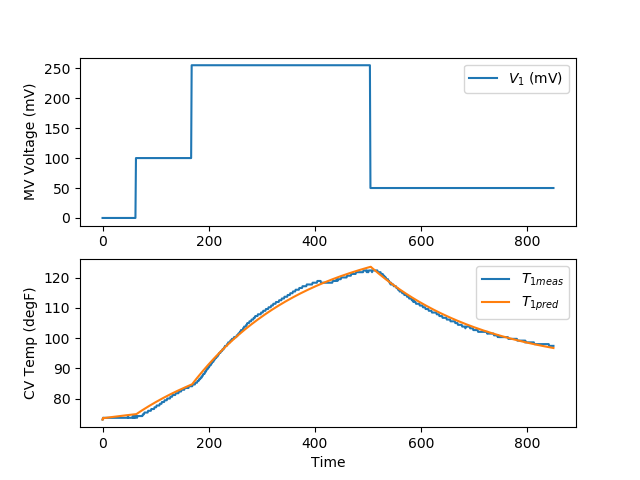(:toggle hide gekko_step button show="Python TCLab SISO ID with Step Test Validation":) (:div id=gekko_step:) (:source lang=python:) from gekko import GEKKO import numpy as np import pandas as pd import matplotlib.pyplot as plt

1. load data and parse into columns

url = 'http://apmonitor.com/do/uploads/Main/tclab_siso_data.txt' data = pd.read_csv(url) t = data['time'] u = data['voltage'] y = data['temperature']

1. generate time-series model

m = GEKKO()

1. system identification

na = 2 # output coefficients nb = 2 # input coefficients yp,p,K = m.sysid(t,u,y,na,nb,pred='meas')

plt.figure() plt.subplot(2,1,1) plt.plot(t,u) plt.legend([r'$V_1$ (mV)']) plt.ylabel('MV') plt.subplot(2,1,2) plt.plot(t,y) plt.plot(t,yp) plt.legend([r'$T_{1meas}$',r'$T_{1pred}$']) plt.ylabel('CV') plt.xlabel('Time') plt.savefig('sysid.png')

1. step test model

yc,uc = m.arx(p)

1. steady state initialization

m.options.IMODE = 1 m.solve(disp=False)

1. dynamic simulation (step test)

m.time = np.linspace(0,240,241) m.options.TIME_SHIFT=0 m.options.IMODE = 4 m.solve(disp=False)

1. step for first MV (Heater 1)

uc.value = np.zeros(len(m.time)) uc.value[5:] = 100 m.solve(disp=False)

plt.figure() plt.subplot(2,1,1) plt.title('Step Test 1') plt.plot(m.time,uc.value,'b-',label=r'$H_1$') plt.ylabel('Heater (V)') plt.legend()

plt.subplot(2,1,2) plt.plot(m.time,yc.value,'b--',label=r'$T_1$') plt.ylabel('Temperature (K)') plt.legend() plt.xlabel('Time (sec)') plt.legend() plt.savefig('step_test.png') plt.show() (:sourceend:) (:divend:)January 30, 2018, at 05:56 PM by 174.148.219.85 -
Changed lines 19-20 from:

For this exercise, you can assume that the dead-time (theta) is zero and estimate only the time constant (tau) and gain (K). The following data set was generated in class from a voltage input (u) that changed the temperature (x) of a thermistor from the Arduino Lab.

to:

For this exercise, you can assume that the dead-time (\theta) is zero and estimate only the time constant (\tau) and gain (K). The following data set was generated in class from a voltage input (u) that changed the temperature (x) of a thermistor from the Arduino Lab.

Changed lines 24-25 from:

Fit the dynamic data to a first order linear system by estimating tau (time constant) and K (gain). Determine the 95% and 99% confidence intervals for the parameters (see F-test video tutorial).

to:

Fit the dynamic data to a first order linear system by estimating \tau (time constant) and K (gain). Determine the 95% and 99% confidence intervals for the parameters (see F-test video tutorial).

Changed line 62 from:

with p=2 (number of parameters), n=851 (number of measurements), theta=[K,tau] (parameters), theta* as the optimal parameters, SSE as the sum of squared errors, and the F statistic that has 3 arguments (alpha=confidence level, degrees of freedom 1, and degrees of freedom 2). For many problems, this creates a multi-dimensional nonlinear confidence region. In the case of 2 parameters, the nonlinear confidence region is a 2-dimensional space.

to:

with p=2 (number of parameters), n=851 (number of measurements), \theta=[K,\tau] (parameters), \theta^* as the optimal parameters, SSE as the sum of squared errors, and the F statistic that has 3 arguments (alpha=confidence level, degrees of freedom 1, and degrees of freedom 2). For many problems, this creates a multi-dimensional nonlinear confidence region. In the case of 2 parameters, the nonlinear confidence region is a 2-dimensional space.

January 30, 2018, at 05:54 PM by 174.148.219.85 -
Changed line 60 from: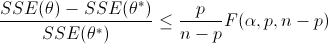to:

$$\frac{SSE(\theta)-SSE(\theta^*)}{SSE(\theta^*)}\le \frac{p}{n-p} F\left(\alpha,p,n-p\right)$$

Changed line 30 from:

$$x_k = \left(x_{k-1}-x_0\right) e^{-\frac{-\Delta t}{\tau}} + \left(u_{k-1}-u_0\right)K\left(1-e^{-\frac{-\Delta t}{\tau}}\right) + x_0$$

to:

$$x_k = \left(x_{k-1}-x_0\right) e^{-\Delta t/\tau} + \left(u_{k-1}-u_0\right)K\left(1-e^{-\Delta t/\tau}\right) + x_0$$

Changed line 30 from:

{$x_k = \left(x_{k-1}-x_0\right) e^{-\frac{-\Delta t}{\tau}} + \left(u_{k-1}-u_0\right)K\left(1-e^{-\frac{-\Delta t}{\tau}}\right) + x_0} to: $$x_k = \left(x_{k-1}-x_0\right) e^{-\frac{-\Delta t}{\tau}} + \left(u_{k-1}-u_0\right)K\left(1-e^{-\frac{-\Delta t}{\tau}}\right) + x_0$$ Changed lines 30-33 from: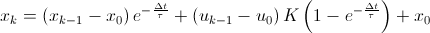In this equation, the subscripts denote the sampling intervals that are collected at a time interval of delta time = tk-tk-1. The above equation and the data are put into an Excel worksheet. The difference between the measured and predicted values are minimized to obtain the optimal parameters K (gain) and tau (time constant). to: {$x_k = \left(x_{k-1}-x_0\right) e^{-\frac{-\Delta t}{\tau}} + \left(u_{k-1}-u_0\right)K\left(1-e^{-\frac{-\Delta t}{\tau}}\right) + x_0}

In this equation, the subscripts denote the sampling intervals that are collected at a time interval of delta time = tk-tk-1. The above equation and the data are put into an Excel worksheet. The difference between the measured and predicted values are minimized to obtain the optimal parameters K (gain) and \tau (time constant).

Changed line 45 from:

An alternative approach to the sum of squared errors is to minimize an l1-norm error to find the optimal values of K (gain) and tau (time constant).

to:

An alternative approach to the sum of squared errors is to minimize an l1-norm error to find the optimal values of K (gain) and \tau (time constant).

Changed lines 5-7 from:

Many Single Input, Single Output (SISO) dynamic systems can be represented empirically with a First Order Plus Dead Time (FOPDT) model. Model identification involves fitting parameters in a dynamic continuous or discrete form of the FOPDT model. The unknown parameters for this system include the time constant (tau), gain (K), and sometimes dead-time (theta).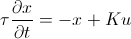to:

Many Single Input, Single Output (SISO) dynamic systems can be represented empirically with a First Order Plus Dead Time (FOPDT) model. Model identification involves fitting parameters in a dynamic continuous or discrete form of the FOPDT model. The unknown parameters for this system include the time constant (\tau), gain (K), and sometimes dead-time (\theta).

$$\tau \frac{dx}{dt} = -x + K \, u\left(t-\theta\right)$$

Changed line 19 from:

For this exercise, you can assume that the dead-time (theta) is zero and estimate only the time constant (tau) and gain (K). The following data set was generated in class from a voltage input (u) that changed the temperature (x) of a thermister.

to:

For this exercise, you can assume that the dead-time (theta) is zero and estimate only the time constant (tau) and gain (K). The following data set was generated in class from a voltage input (u) that changed the temperature (x) of a thermistor from the Arduino Lab.

Changed line 3 from:

(:description Single Input, Single Output model identification from dynamic data.:)

to:

(:description Single Input, Single Output model identification from dynamic data with example solutions in Excel, MATLAB, and Python.:)

Changed line 3 from:

(:description Simulink (MATLAB) tutorial for adopting Differential Algebraic Equation (DAE) systems in a model for dynamic simulation, estimation, and control:)

to:

(:description Single Input, Single Output model identification from dynamic data.:)

Changed line 2 from:

(:keywords dynamic data, simulation, validation, differential, algebraic, tutorial, Simulink:)

to:

(:keywords dynamic data, model identification, validation, differential, algebraic, tutorial, Simulink:)

Changed line 5 from:

Dynamic estimation involves fitting parameters in a dynamic model. One example of an empirical model is a linear first order differential equation that can approximate the dynamic evolution of many simple systems. The unknown parameters for this system include the time constant (tau), gain (K), and sometimes dead-time (theta).

to:

Many Single Input, Single Output (SISO) dynamic systems can be represented empirically with a First Order Plus Dead Time (FOPDT) model. Model identification involves fitting parameters in a dynamic continuous or discrete form of the FOPDT model. The unknown parameters for this system include the time constant (tau), gain (K), and sometimes dead-time (theta).

Changed line 1 from:

(:title Dynamic Data Simulation:)

to:

(:title SISO Model Identification:)

May 06, 2015, at 01:27 PM by 45.56.3.184 -
Deleted lines 8-14:

For this exercise, you can assume that the dead-time (theta) is zero and estimate only the time constant (tau) and gain (K). The following data set was generated in class from a voltage input (u) that changed the temperature (x) of a thermister.Download Dynamic Data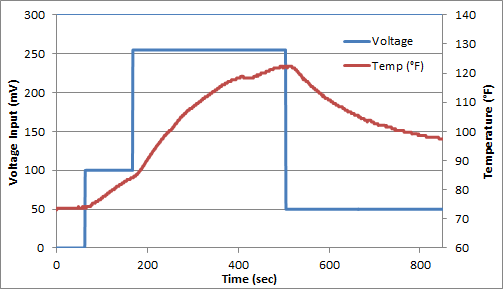Fit the dynamic data to a first order linear system by estimating tau (time constant) and K (gain). Determine the 95% and 99% confidence intervals for the parameters (see F-test video tutorial).

Changed lines 17-24 from:

to:

#### Exercise

For this exercise, you can assume that the dead-time (theta) is zero and estimate only the time constant (tau) and gain (K). The following data set was generated in class from a voltage input (u) that changed the temperature (x) of a thermister.Download Dynamic DataFit the dynamic data to a first order linear system by estimating tau (time constant) and K (gain). Determine the 95% and 99% confidence intervals for the parameters (see F-test video tutorial).

May 06, 2015, at 01:15 PM by 45.56.3.184 -

#### Model/Process Mismatch

May 06, 2015, at 01:11 PM by 45.56.3.184 -
Changed line 43 from:

An alternative approach to the sum of squared errors is to minimize an L1-norm error to find the optimal values of K (gain) and tau (time constant).

to:

An alternative approach to the sum of squared errors is to minimize an l1-norm error to find the optimal values of K (gain) and tau (time constant).

May 06, 2015, at 01:09 PM by 45.56.3.184 -

The time constant and gain may be incorrect because of system nonlinearity, bad data, or process drift over time. If the model is used in a model predictive controller, a model gain that is lower than the actual process gain may cause controller oscillation and instability. A model gain that is higher than the actual process may cause sluggish control. Likewise, a model response (time constant) that is slow (higher time constant) than the actual process time constant tends to cause controller instability. On the other hand, a model response (time constant) that is fast (lower time constant) than the actual process tends to cause sluggish controller response. This is illustrated by the following objective function plot that shows model predictive control performance with model mismatch to the process.The combination of a low gain and high time constant leads to the highest objective function (poor performance and instability). On the other hand, a high gain and low time constant lead to sluggish control but the controller is able to asymptotically drive the process to the correct set point.

April 30, 2015, at 11:06 PM by 45.56.3.184 -
Changed line 11 from:
to:Download Dynamic Data
Changed line 26 from:
to:Download Excel Worksheet for Dynamic Parameter Estimation
Changed line 39 from:
to:Download MATLAB and Python Solutions for Dynamic Parameter Estimation
Changed line 42 from:

The MATLAB and Python plots produce trends that show the optimal solution with an L1-norm objective function.

to:

The MATLAB and Python plots produce trends that show the optimal solution with an l1-norm objective function.

April 30, 2015, at 10:58 PM by 45.56.3.184 -

Dynamic estimation involves fitting parameters in a dynamic model. One example of an empirical model is a linear first order differential equation that can approximate the dynamic evolution of many simple systems. The unknown parameters for this system include the time constant (tau), gain (K), and sometimes dead-time (theta).For this exercise, you can assume that the dead-time (theta) is zero and estimate only the time constant (tau) and gain (K). The following data set was generated in class from a voltage input (u) that changed the temperature (x) of a thermister.Fit the dynamic data to a first order linear system by estimating tau (time constant) and K (gain). Determine the 95% and 99% confidence intervals for the parameters (see F-test video tutorial).

#### Excel Solution

The dynamic model can be written in discrete form by specifying that the voltage is constant between sampling intervals. The discrete form of the equation accounts for non-zero initial conditions in temperature (x0) and voltage (u0). In this case, the voltage does start at zero, but this is not required.In this equation, the subscripts denote the sampling intervals that are collected at a time interval of delta time = tk-tk-1. The above equation and the data are put into an Excel worksheet. The difference between the measured and predicted values are minimized to obtain the optimal parameters K (gain) and tau (time constant).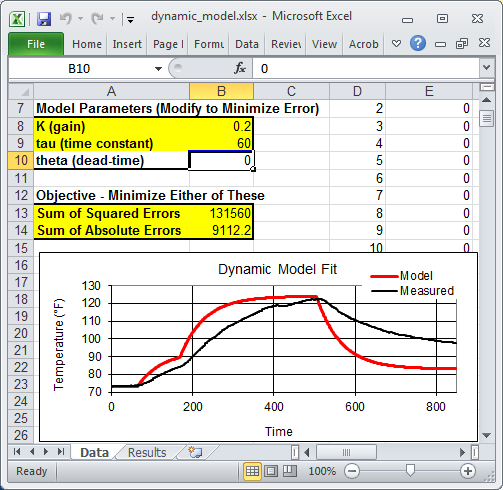The spreadsheet is currently showing non-optimal values. To obtain the optimal values, use Excel Solver available from the Data toolbar. If the solver option does not appear, it can be added with the Add-In manager.#### MATLAB and Python Solutions

An alternative approach to the sum of squared errors is to minimize an L1-norm error to find the optimal values of K (gain) and tau (time constant).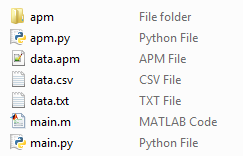The MATLAB and Python plots produce trends that show the optimal solution with an L1-norm objective function.The nonlinear confidence interval is shown by producing a contour plot of the SSE objective function with variations in the two parameters. The optimal solution is shown at the center of the plot and the objective function becomes worse (higher) away from the optimal solution.The confidence interval is determined with an F-test that specifies an upper limit to the deviation from the optimal solutionwith p=2 (number of parameters), n=851 (number of measurements), theta=[K,tau] (parameters), theta* as the optimal parameters, SSE as the sum of squared errors, and the F statistic that has 3 arguments (alpha=confidence level, degrees of freedom 1, and degrees of freedom 2). For many problems, this creates a multi-dimensional nonlinear confidence region. In the case of 2 parameters, the nonlinear confidence region is a 2-dimensional space.